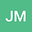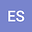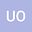loading page

Global attractors, extremal stability and periodicity for a delayed population model with survival rate on isolated time scales
•••• +1
• Jaqueline Godoy Mesquita,
• Ewa Schmeidel,
• Urszula Ostaszewska,
• Malgorzata Zdanowicz
Jaqueline Godoy Mesquita
Universidade de Brasilia
Author ProfileEwa Schmeidel
University of Bialystok, Poland
Author ProfileUrszula Ostaszewska
University of Bialystok
Author ProfileMalgorzata Zdanowicz
University of Bialystok, Poland
Author Profile## Abstract

In this paper, we investigate the existence of global attractors, extreme stability, periodicity and asymptotically periodicity of solutions of the delayed population model with survival rate on isolated time scales given by $x^{\Delta} (t) = \gamma(t) x(t) + \dfrac{x(d(t))}{\mu(t)}e^{r(t)\mu(t)\left(1 - \frac{x(d(t))}{\mu(t)}\right)}, \ \ t \in \mathbb T.$ We present many examples to illustrate our results, considering different time scales